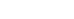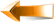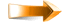# RealVol Daily Indices

• The Flagship index at RealVol is the 1-month (21-day) realized volatility index (also known as VOLm)
• Detailed explanations can be found at the bottom of the page

VOLS: RealVol SPY Indices

 VOLSPYHistoricalSymbol 1-Year ½-Year 1-Quarter 1-Month 1-Week 1-Day VOL 21.39 26.84 29.90 26.22 29.73 41.37 VOV 8.98 11.12 18.57 70.63 475.64 1705.95 DVOL 19.57 24.61 27.49 23.45 27.74 30.81 VCOR -25.25 -28.76 -20.92 -1.80 n/a n/a VAR 457.53 720.39 894.01 687.49 883.87 1711.23ForecastSymbol 1-Day 1-Week 1-Month 1-Quarter ½-Year 1-Year RVOL 27.08 26.52 25.66 24.96 24.55 24.22 HVOL 20.59 19.95 19.42 18.15 17.03 16.10
 As of: 03 Oct 2022 Charts Underlying Asset

Updates at ≈12:30 A.M. (preliminary) and ≈4:30 A.M. (final) ET Tuesday through Saturday
Source of Underlying Data: NYSE Arca

### Color Key

 VOLd™ 1-day RealVol Index of realized volatility (using RealVol Daily Formula) VOLw™ 1-week (5-day) RealVol Index of realized volatility (using RealVol Daily Formula) VOLm™ 1-month (21-day) RealVol Index of realized volatility (using RealVol Daily Formula) VOLq™ 1-quarter (63-day) RealVol Index of realized volatility (using RealVol Daily Formula) VOLh™ ½-year (126-day) RealVol Index of realized volatility (using RealVol Daily Formula) VOLy™ 1-year (252-day) RealVol Index of realized volatility (using RealVol Daily Formula) VOVd™ 21-day of 1-day RealVol Index (vol of vol) (using RealVol Daily Formula) VOVw™ 21-day of 1-week (5-day) RealVol Index (vol of vol) (using RealVol Daily Formula) VOVm™ 21-day of 1-month (21-day) RealVol Index (vol of vol) (using RealVol Daily Formula) VOVq™ 21-day of 1-quarter (63-day) RealVol Index (vol of vol) (using RealVol Daily Formula) VOVh™ 21-day of ½-year (126-day) RealVol Index (vol of vol) (using RealVol Daily Formula) VOVy™ 21-day of 1-year (252-day) RealVol Index (vol of vol) (using RealVol Daily Formula) DVOLd™ 1-day RealVol Index of overnight/intraday realized volatility (using RealVol Overnight/Intraday Formula) DVOLw™ 1-week (5-day) RealVol Index of overnight/intraday realized volatility (using RealVol Overnight/Intraday Formula) DVOLm™ 1-month (21-day) RealVol Index of overnight/intraday realized volatility (using RealVol Overnight/Intraday Formula) DVOLq™ 1-quarter (63-day) RealVol Index of overnight/intraday realized volatility (using RealVol Overnight/Intraday Formula) DVOLh™ ½-year (126-day) RealVol Index of overnight/intraday realized volatility (using RealVol Overnight/Intraday Formula) DVOLy™ 1-year (252-day) RealVol Index of overnight/intraday realized volatility (using RealVol Overnight/Intraday Formula) VCORd™ N/A VCORw™ N/A VCORm™ 1-month (21-day) RealVol Index of historical correlation based on the underlying and VOLm (using RealVol Correlation Formula) VCORq™ 1-quarter (63-day) RealVol Index of historical correlation based on the underlying and VOLq (using RealVol Correlation Formula) VCORh™ ½-year (126-day) RealVol Index of historical correlation based on the underlying and VOLh (using RealVol Correlation Formula) VCORy™ 1-year (252-day) RealVol Index of historical correlation based on the underlying and VOLy (using RealVol Correlation Formula) VARd™ 1-day RealVol Index of realized variance (using RealVol Variance Formula) VARw™ 1-week (5-day) RealVol Index of realized variance (using RealVol Variance Formula) VARm™ 1-month (21-day) RealVol Index of realized variance (using RealVol Variance Formula) VARq™ 1-quarter (63-day) RealVol Index of realized variance (using RealVol Variance Formula) VARh™ ½-year (126-day) RealVol Index of realized variance (using RealVol Variance Formula) VARy™ 1-year (252-day) RealVol Index of realized variance (using RealVol Variance Formula) RVOLd™ 1-day RealVol Index of forecast realized volatility (using RFSV model) RVOLw™ 1-week (5-day) RealVol Index of forecast realized volatility (using RFSV model) RVOLm™ 1-month (21-day) RealVol Index of forecast realized volatility (using RFSV model) RVOLq™ 1-quarter (63-day) RealVol Index of forecast realized volatility (using RFSV model) RVOLh™ ½-year (126-day) RealVol Index of forecast realized volatility (using RFSV model) RVOLy™ 1-year (252-day) RealVol Index of forecast realized volatility (using RFSV model) HVOLd™ 1-day RealVol Index of forecast realized volatility (using HARK model) HVOLw™ 1-week (5-day) RealVol Index of forecast realized volatility (using HARK model) HVOLm™ 1-month (21-day) RealVol Index of forecast realized volatility (using HARK model) HVOLq™ 1-quarter (126-day) RealVol Index of forecast realized volatility (using HARK model) HVOLh™ ½-year (126-day) RealVol Index of forecast realized volatility (using HARK model) HVOLy™ 1-year (252-day) RealVol Index of forecast realized volatility (using HARK model) * An asterisk indicates that the data are not yet available.